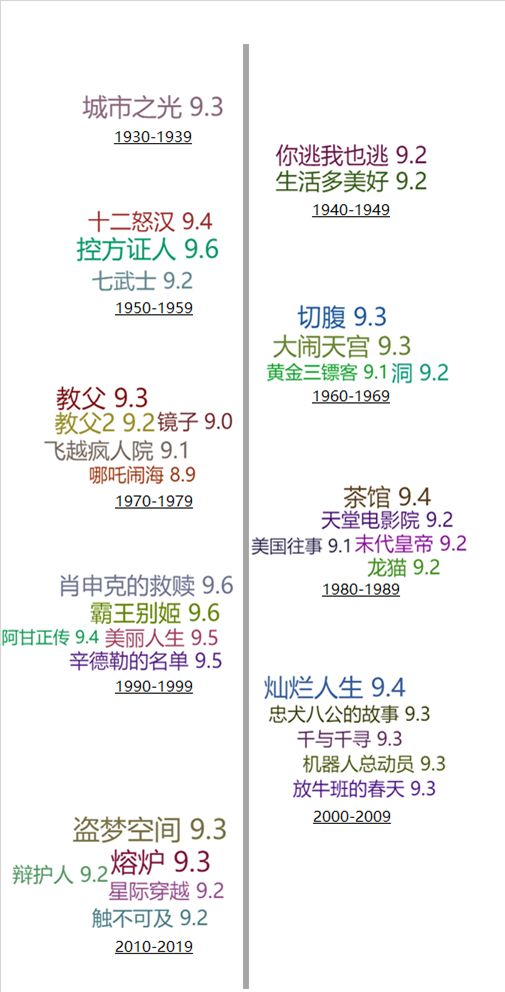摆脱剧荒！教你用 Python 一步步爬取豆瓣电影新榜单1、网址规律探究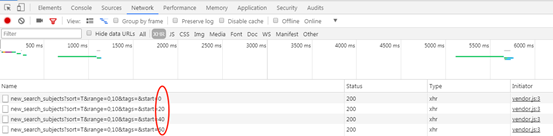2、单页解析+循环爬取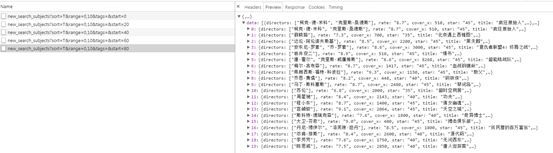headers= { 'User-Agent': 'Mozilla/5.0 (Windows NT 6.1; WOW64) AppleWebKit/537.36 (KHTML, like Gecko) Chrome/63.0.3239.132 Safari/537.36'}

df = pd.DataFrame

fori inbs[ 'data']:

casts = i[ 'casts'] #主演

cover = i[ 'cover'] #海报

directors = i[ 'directors'] #导演

m_id = i[ 'id'] #ID

rate = i[ 'rate'] #评分

star = i[ 'star'] #标记人数

title = i[ 'title'] #片名

url = i[ 'url'] #网址

cache = pd.DataFrame({ '主演':[casts], '海报':[cover], '导演':[directors],

'ID':[m_id], '评分':[rate], '标记':[star], '片名':[title], '网址':[url]})

df = pd.concat([df,cache])

returndf

#你想爬取多少页，其实这里对应着加载多少次

defformat_url(num):

urls = []

base_url = 'https://movie.douban.com/j/new_search_subjects?sort=T&range=0,10&tags=%E7%94%B5%E5%BD%B1&start={}'

fori inrange( 0, 20* num, 20):

url = base_url.format(i)

urls.append(url)

returnurls

urls = format_url( 450)

result = pd.DataFrame

#看爬取了多少页

count = 1

forurl inurls:

result = pd. concat([result,df])

time.sleep( random. random+ 2)

print( 'I had crawled page of:%d'% count)

count += 13、单部电影详情爬取：ifip == '':

else:

html = requests.get(url,headers = headers,proxies = ip)

bs = etree.HTML(html.text)

#片名

title = bs.xpath( '//div[@id = "wrapper"]/div/h1/span')[ 0].text

#上映时间

year = bs.xpath( '//div[@id = "wrapper"]/div/h1/span')[ 1].text

#电影类型

m_type = []

fort inbs.xpath( '//span[@property = "v:genre"]'):

m_type.append(t.text)

a = bs.xpath( '//div[@id= "info"]')[ 0].xpath( 'string')

#片长

m_time =a[a.find( '片长: ') + 4:a.find( '分钟n')] #时长

#地区

area = a[a.find( '制片国家/地区:') + 9:a.find( 'n 语言')] #地区

#评分人数

try:

people = bs.xpath( '//a[@class = "rating_people"]/span')[ 0].text

#评分分布

rating = {}

rate_count = bs.xpath( '//div[@class = "ratings-on-weight"]/div')

forrate inrate_count:

rating[rate.xpath( 'span/@title')[ 0]] = rate.xpath( 'span[@class = "rating_per"]')[ 0].text

except:

people = 'None'

rating = {}

#简介

try:

brief = bs.xpath( '//span[@property = "v:summary"]')[ 0].text.strip( 'n u3000u3000')

except:

brief = 'None'

try:

hot_comment = bs.xpath( '//div[@id = "hot-comments"]/div/div/p/span')[ 0].text

except:

hot_comment = 'None'

cache = pd.DataFrame({ '片名':[title], '上映时间':[year], '电影类型':[m_type], '片长':[m_time],

'地区':[area], '评分人数':[people], '评分分布':[rating], '简介':[brief], '热评':[hot_comment], '网址':[url]})

returncache

PS：代理IP如果展开讲篇幅太长，网上有许多免费的IP代理（缺点是可用时间短，不稳定）和付费的IP代理（缺点是不免费）。另外，要强调一下这里我们传入的IP长这样：{'https':'https://115.219.79.103:0000'}

movie_result = pd.DataFrame

ip = ''#这里构建自己的IP池

count2 = 1

cw = 1

forurl,name inzip(result[ '网址'].values[ 6000:],result[ '片名'].values[ 6000:]):

# forname,url inwrongs.items:

try:

cache = parse_movie_info(url,headers = headers,ip = ip)

movie_result = pd. concat([movie_result,cache])

# time.sleep( random. random)

print( '我们爬取了第:%d部电影-------%s'% (count2,name))

count2 += 1

except:

print( '滴滴滴滴滴，第{}次报错'. format(cw))

print( 'ip is:{}'. format(ip))

cw += 1

time.sleep( 2)

continue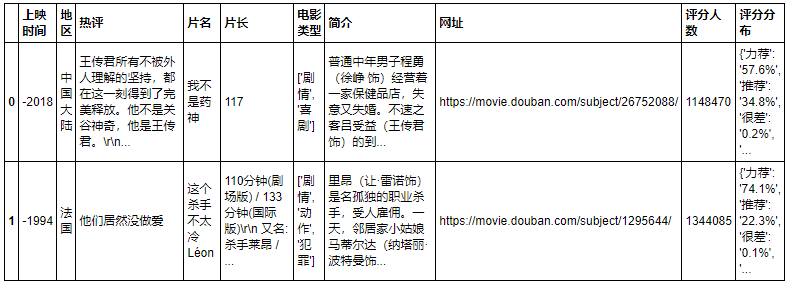1、基本信息表和电影内容表合并

base_info表里面是我们批量抓取的电影基本信息，movie_info则是我们进入每一部电影，获取到的感兴趣字段汇总，后面的分析是需要依赖两张表进行的，所以我们合并之：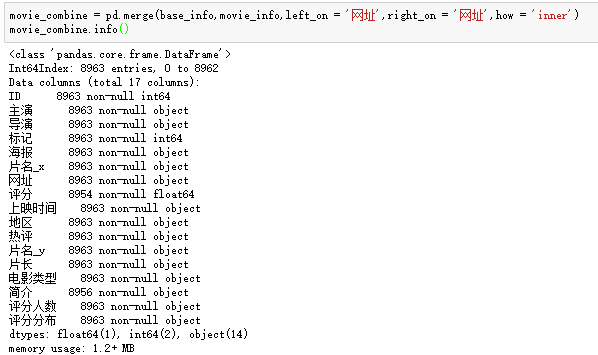2、电影年份数据清洗：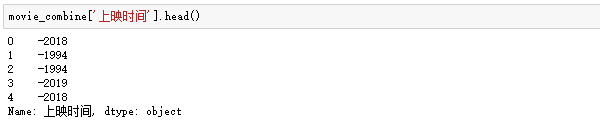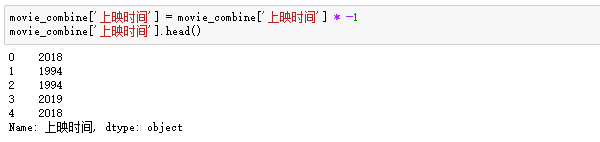3、评分分布规整：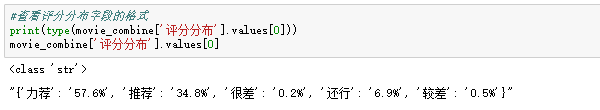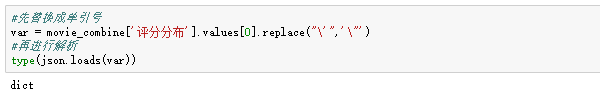#把单列字典的评分分布转化成分开的5列，且每一列是数值型的

defget_rate(x,types):

try:

returnfloat(x[types].strip( '%'))

except:

pass

movie_combine[ '5星'] = movie_combine[ 'format_评分'].apply(get_rate,types = '力荐')

movie_combine[ '4星'] = movie_combine[ 'format_评分'].apply(get_rate,types = '推荐')

movie_combine[ '3星'] = movie_combine[ 'format_评分'].apply(get_rate,types = '还行')

movie_combine[ '2星'] = movie_combine[ 'format_评分'].apply(get_rate,types = '较差')

movie_combine[ '1星'] = movie_combine[ 'format_评分'].apply(get_rate,types = '很差')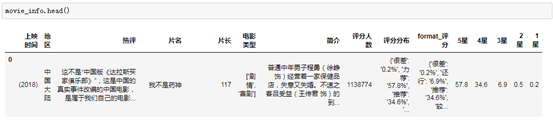OK，清洗到此告一段落。GET到这个点，我们就可以对电影评分排序制定一个简单的规则：先按照总评分排序，然后再对比5星人数占比，如果一样就对比4星，以此类推。这个评分排序逻辑用PYTHON做起来不要太简单，一行代码就搞定：

#按照总评分，5星评分人数占比，4星占比，3星..依次类推

movie_combine.sort_values([ '评分', '5星', '4星', '3星', '2星', '1星'],ascending = False,inplace = True)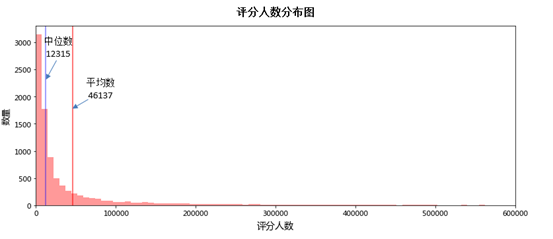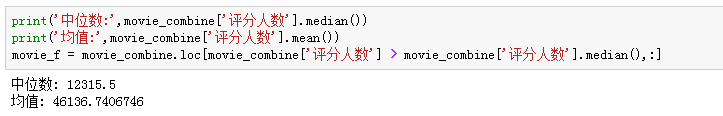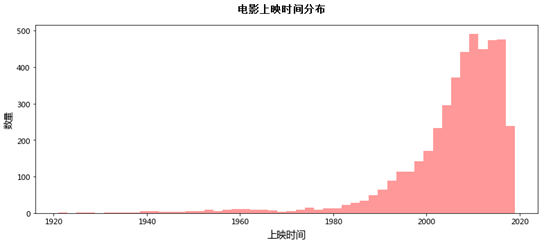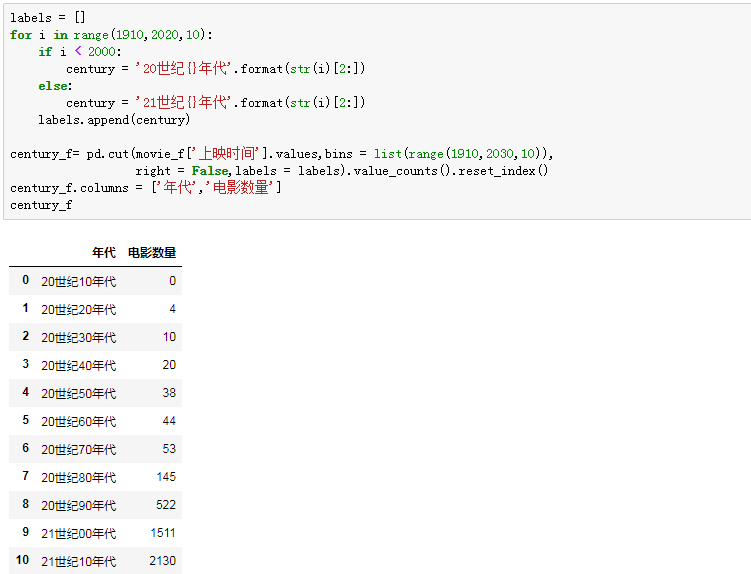• 基于年代上映量数据，我们从20世纪30年代开始制作排名；
• 为了避免有些年代电影过少，优化成各年代TOP 10%的电影推荐；
• 同时，为了避免近年电影过多，每个年代推荐的上限数不超过100部。

final_rank = pd.DataFrame

forcentury, count inzip(century_f.index,century_f.values):

f1 = movie_f2.loc[movie_f[ '年代'] == century,:]

#1000部以下的，取TOP10%

ifcount < 1000:

return_num = int(count * 0.1)

#1000部以上的，取前100部

else:

return_num = 100

f2 = f1.iloc[:return_num,:]

final_rank = pd.concat([final_rank,f2])